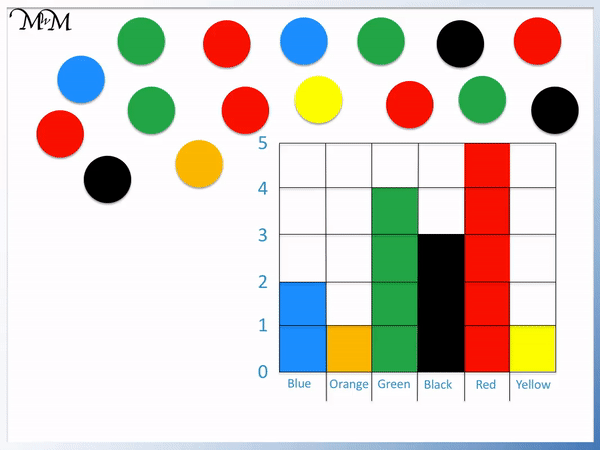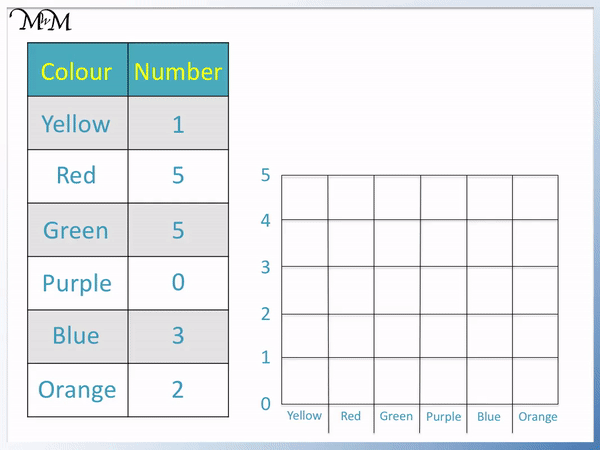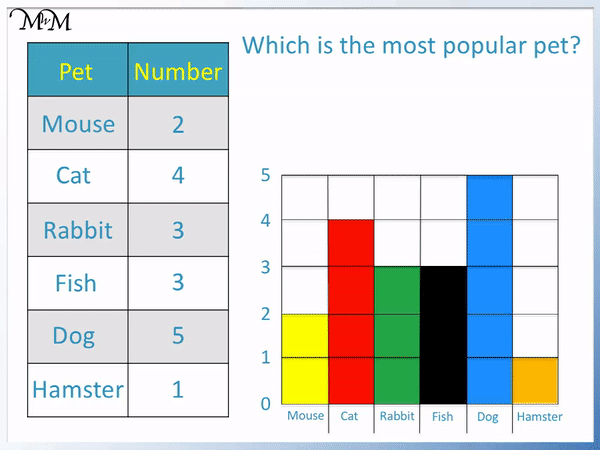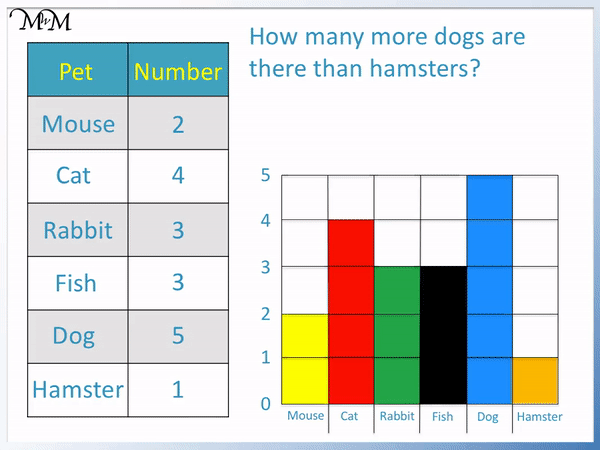# Block Graphs / Block Diagrams

Block Graphs / Block Diagrams• A block graph (also known as a block diagram) is a simple way to present data with coloured squares.
• Each block represents one item.
• The types of each object is written along the bottom of the graph and the number of each object is written along the side.
• The number of blocks in each column tell us how many of each colour there are.
• The top of the blocks line up with the number of counters of that colour.

• Block diagrams are a useful introduction to bar charts.
• Block diagrams are different from bar charts in that each square on a block diagram is always worth 1 item and there are no gaps between the bars.

Each coloured square is worth one item.

The number of blocks in each column of a block graph tell us how many there are of that object.• We will draw a block graph to show the favourite colours of children in a class.
• The table shows the number of children who have each colour as their favourite.
• The number that is next to each colour in the table will be the number of blocks that are shaded in in each column of the block graph.
• We can see that both green and red have 5 blocks shaded in and are the same height.
• Because zero people prefer the colour purple, no blocks are drawn in this column.#### Block Graphs: Accompanying Lesson Worksheet# What are Block Graphs / Block Diagrams?

Block diagrams are also known as block graphs. Block diagrams are one of the first methods for presenting data that a child will see in school. Block diagrams are often introduced before teaching bar charts in key stage 1 (or first grade).

A block graph is drawn on square grid paper. In a block graph the different categories are listed along the bottom of the graph below each square on the grid. The number of blocks in each category tell us how many of each category there are.

A block graph looks similar to a bar chart but it is different in a couple of ways. A block graph does not have spaces in between each category like a bar chart does. Each shaded block on a block graph is always worth one, whereas on a bar chart this is not the case. On a bar chart, each block value depends on the scale of the chart. On a bar chart the blocks are not separate and instead form one solid bar.

Sometimes, a block graph may contain images of items rather than a shaded in square, almost like a pictogram. However, in this lesson, as is the case usually, each block will be shaded in.

Here is our first example of using a block diagram.

In this example we will draw a block graph to show the number of each colour counter.

We start by writing the names of the colours below each square on the bottom of the block diagram.Each counter is worth one block.

We can take away each counter as we count it so that we do not count it twice.

As we pick up and count each counter, another block is shaded in.Each block is worth one counter. When teaching block graphs, it can be helpful to use different colours for each category.

In this example we are using the same colour blocks as counters.

To read a block diagram, read the number which is in line with the top of each set of blocks.

It may be helpful to use a ruler. You can line the ruler up with the top of the block and read across it to see the number.

In this example, we have a table showing the favourite colours of children in a class.

We will use this table to draw a block graph.The number of children that prefer each colour is written next to the colour in the table.

The number next to each colour is the number of blocks that need drawing.

In this example, we are given a block graph and will use it to complete the table.

This block graph shows the number of pets owned by children in a class.The mouse column contains 2 blocks. The top of the block is in line with a 2.

This means that there are 2 mice owned by children in this class. We write a 2 in the table next to ‘mice’.

There are 4 blocks in the cat column of the block diagram. The top of this set of blocks is in line with 4.

This means that there are 4 cats owned by children in this class.

There are 3 rabbits and 3 fish.

There are 5 dogs owned in this class. This is the tallest set of blocks.

There is only 1 hamster owned in this class. This is the shortest set of blocks.

When teaching children to read block diagrams, you can start by counting the number of blocks in each column but it is worth encouraging them to simply read off the number that the top of each set of blocks is in line with.

This skill follows through into bar charts and other reading other graphs later in school life. It is also much quicker.

Identifying the longest and shortest set of blocks is also worth doing.

You will often be asked to interpret information from block diagrams.

Here is an example of a question interpreting a block graph.

Which is the most popular pet?The most popular pet is the pet that is owned by more children than any other pet.

It will be the pet that has the most blocks in its column.

The dog has 5 blocks and so, it is the most popular pet. The dog column is the tallest because it has the most blocks.

Here is another question interpreting data from this block graph.

What is the least popular pet?The hamster is the least popular pet because it has the fewest blocks. The hamster has the shortest set of blocks.

Only 1 person has a hamster.

In this question we are asked what is the difference between the number of dogs and the number of hamsters.

How many more dogs are there than hamsters?

We can count how many blocks are in each and find the difference.

There are 5 dogs.

There is 1 hamster.

We can count upwards on the block graph to see how many more we need to get to 5 from 1.There are 4 more dogs than hamsters.

5 is 4 more than 1.

To find the difference in maths, we can use subtraction. Subtracting the smaller number from the bigger number tells us the difference between the numbers.

5 – 1 = 4 and so, there are 4 more dogs than hamsters.Now try our lesson on Drawing and Interpreting Bar Charts where we learn how to plot bar charts to display data.